# Acids

70% acid made from the same acid of two different concentrations. Amount weaker acid to the stronger acid is in ratio 2:1.

What was the concentration of the weaker acid if stronger had 91% concentration?

c =  59.5 %

### Step-by-step explanation: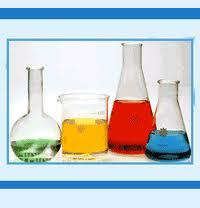Did you find an error or inaccuracy? Feel free to write us. Thank you!Tips to related online calculators
Do you have a linear equation or system of equations and looking for its solution? Or do you have a quadratic equation?

## Related math problems and questions:

• Acid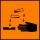The barrel is 199 liters of 38% acid. How many percent acids occur when 61 liters revoke and replace with the same amount of water?
• Acid solutionBy adding 250 grams of a 96% sulfuric acid solution to its 3% solution, we changed its initial concentration to 25%. How many grams of 3% of the acid was used for dilution?
• ConcentrationHow many grams of 65% and 50% acid is to be mixed to give 240 g acid with a concentration of 60%?
• Acid evaporationHow many kilograms of water do we have to evaporate from 100 kg of 32% acid to make it 80% concentration?
• Hydrochloric acidDetermine the concentration of which must have a solution of hydrochloric acid that mixing 10 l of the solution with 8 liters of 26% solution to get the solution with a concentration of 50%?
• AlcoholHow many 55% alcohol we need to pour into 14 liters 75% alcohol to get p3% alcohol? How many 65% alcohol we get?
• Concentration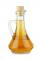How 0.5 liters of 8% vinegar diluted to a concentration of 20 hundredths % of vinegar? How many liters of water must be poured?
• Solutions, mixturesHow many liters of 70% solution we must add to 5 liters of 30% solution to give us a 60% solution?
• Solutions, mixturesWe have 2 liters of 20% solution available. How much 70% solution do we need to add to it to get a 30% solution?
• Vinegar 2How many percentages of vinegar solution will we get if we mix 3.5 liters of 5.8% and 5 liters of 7.6% vinegar?
• Alcohol mixing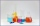How much 64% of the alcohol must be poured into 6 liters of 85% alcohol to produce 79% alcohol?
• Vinegar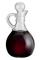We must dilute 16 liters of 8.8% aqueous vinegar to 4.1% one. How much water is necessary to add?
• Alcohol 2Two types of alcohol one 63% and second 75% give 20 liters of 69% alcohol. How many liters of each type are in the mixture?
• Pouring alcohol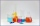100 liters of alcohol has 70% How many liters of water need to be added to have 60% alcohol?
• SolutionWhat amount of 80% solution and what amount of water is needed to mix in order to obtain two liters of 60% solution of this mixture?
• Alcohol mixtureThree liters of 96 percent alcohol set up a certain amount of distilled water to form 54-percent alcohol. How many liters of distilled water was used?
• Mushrooms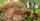Fresh mushrooms contain 90% water, dried only 12%. How many kilograms of fresh mushrooms are needed for 5kg dried?